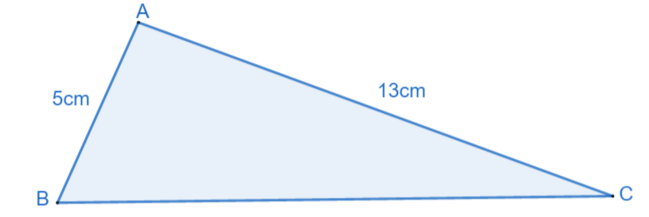# Exam-Style Questions.

## Problems adapted from questions set for previous Mathematics exams.

### 1.

IB Analysis and Approaches

In triangle ABC, the length of AB = 5cm, AC = 13cm and $$\cos B \hat AC = \frac18$$.

(a) find the value of $$\sin B \hat AC$$

(b) Find the area of triangle ABC.Diagram not to scale

### 2.

IB Standard

Consider a right-angled triangle, ABC, with the right angle at vertex C and where $$\sin A = \frac{12}{13}$$

(a) Show that $$\cos A = \frac{5}{13}$$

(b) Find $$\sin 2A$$.

### 3.

A-Level

The cosine of acute angle $$\alpha$$ is $$\frac{1}{ \sqrt 5}$$

The angle $$\beta$$ is obtuse and $$\sin \beta = \sqrt \frac{2}{3}$$.

(a) Find exact values of $$\tan \alpha$$ and $$\tan \beta$$.

(b) Hence show that $$\tan( \alpha - \beta )$$ can be written as $$a+b \sqrt 2$$ where $$a$$ and $$b$$ are rational numbers

The exam-style questions appearing on this site are based on those set in previous examinations (or sample assessment papers for future examinations) by the major examination boards. The wording, diagrams and figures used in these questions have been changed from the originals so that students can have fresh, relevant problem solving practice even if they have previously worked through the related exam paper.

The solutions to the questions on this website are only available to those who have a Transum Subscription.

Exam-Style Questions Main Page

Search for exam-style questions containing a particular word or phrase:

To search the entire Transum website use the search box in the grey area below.